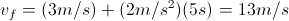# MCAT Physical : Motion in One Dimension

## Example Questions

← Previous 1 3

### Example Question #1 : Motion In One Dimension

A 2kg mass is suspended on a rope that wraps around a frictionless pulley attached to the ceiling with a mass of 0.01kg and a radius of 0.25m. The other end of the rope is attached to a massless suspended platform, upon which 0.5kg weights may be placed. While the system is initially at equilibrium, the rope is later cut above the weight, and the platform subsequently raised by pulling on the rope.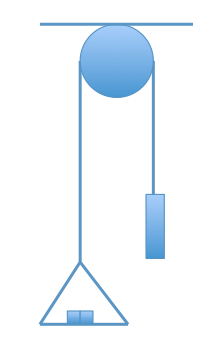The rope is cut right above the 2 kgmass. What is the acceleration of the platform with the individual weights as it falls to the ground?

19.6m/s2

9.8m/s2

4.9m/s2

0m/s2

9.8m/s2

Explanation:

Remember that Newton’s second law is applicable regardless of whether we are talking about kinematics, torque, electric, magnetic, or gravitational force, thus, F = ma. We know that the only force acting in free-fall in an environment where we can neglect air resistance (i.e. most of the problems on the MCAT) is gravity. The acceleration due to gravity is 9.8m/s2. The sign could be + or – depending on how you decided to orient.

### Example Question #2 : Motion In One Dimension

A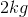ball is dropped off of a roof. If the ball takes four seconds to hit the ground, what is the height from which the ball was dropped?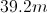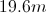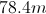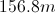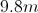Explanation:

We are given the time that the ball is in the air and the acceleration. Since the ball is dropped, we can also tell that the initial velocity is zero (starts from rest). Using these given values, we can calculate the final velocity using the appropriate motion equation.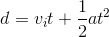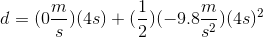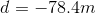The resulting displacement is negative because the ball is traveling downward; however, the height will be the absolute value of the displacement, giving out final answer.

### Example Question #3 : Motion In One Dimension

Aball is dropped off of a roof. If the ball takes four seconds to hit the ground, what is the ball's speed right before it hits the ground?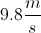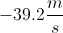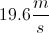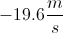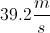Explanation:

We are given the time that the ball is in the air and the acceleration. Since the ball is dropped, we can also tell that the initial velocity is zero (starts from rest). Using these given values, we can calculate the final velocity using the appropriate motion equation.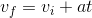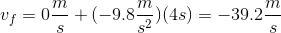The final velocity is negative because the ball is traveling downward; however, the question asks for speed, allowing us to disregard the vector indicator. Speed cannot be negative.

### Example Question #4 : Motion In One Dimension

Suppose a ball with mass of 10kg was dropped from rest from the top of a cliff that is 80m tall. How long will it take the ball to reach the bottom of the cliff?

10s

8s

4s

16s

2s

4s

Explanation:

The question tells us that the initial velocity of the ball (v0) is zero and the height (d) of the cliff is 80m. Acceleration (gravity) is 10m/s2. Using the kinematics eqation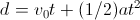we can solve for time.

80 = 0 + (1/2)(10)t2

80/5 = t2 = 16

t = 4s

### Example Question #5 : Motion In One Dimension

An object is shot upward from the ground at a velocity of 24m/s.  How long before it hits the ground?

12.0s

2.4s

4.8s

9.6s

4.8s

Explanation:

First find the time it takes to reach the top of its path using the equation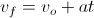, where the final velocity is 0m/s, initial velocity is 24m/s, and a is the acceleration due to gravity.  Solve for time (t).

0 = 24 +(-10)t

t = 2.4s

Notice that this is only the time it takes the object to reach the top of its path, and the question asks for the time it takes the object to reach the ground. In order to find how long it takes to reach the ground simply double this time.

2.4s * 2 = 4.8 s

### Example Question #6 : Motion In One Dimension

Two children are playing on an icy lake. Child 1 weighs 50kg, and child 2 weighs 38kg. Child 1 has a backpack that weighs 10kg, and child 2 has a backpack that weighs 5kg.

Over the course of the afternoon, they collide many times. Four collisions are described below.

Collision 1:

Child 1 starts from the top of a ramp, and after going down, reaches the lake surface while going 5m/s and subsequently slides into a stationary child 2. They remain linked together after the collision.

Collision 2:

Child 1 and child 2 are sliding in the same direction. Child 2, moving at 10m/s, slides into child 1, moving at 2m/s.

Collision 3:

The two children collide while traveling in opposite directions at 10m/s each.

Collision 4:

The two children push off from one another’s back, and begin moving in exactly opposite directions. Child 2 moves with a velocity of +8m/s.

Child 1 hooks up a jet motor to a sled, and brings it to the icy lake. She sets up her sled, and launches from a velocity of +8 m/s with an acceleration of 15m/s2. After ten seconds, how fast is she traveling? Assume the lake is frictionless.

-158m/s

-8m/s

158m/s

150m/s

-150m/s

158m/s

Explanation:

For this question, we have to use the translational motion equations: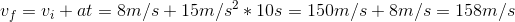### Example Question #1 : Motion In One Dimension

Two children are playing on an icy lake. Child 1 weighs 50kg, and child 2 weighs 38kg. Child 1 has a backpack that weighs 10kg, and child 2 has a backpack that weighs 5kg.

Over the course of the afternoon, they collide many times. Four collisions are described below.

Collision 1:

Child 1 starts from the top of a ramp, and after going down, reaches the lake surface while going 5m/s and subsequently slides into a stationary child 2. They remain linked together after the collision.

Collision 2:

Child 1 and child 2 are sliding in the same direction. Child 2, moving at 10m/s, slides into child 1, moving at 2m/s.

Collision 3:

The two children collide while traveling in opposite directions at 10m/s each.

Collision 4:

The two children push off from one another’s back, and begin moving in exactly opposite directions. Child 2 moves with a velocity of +8m/s.

Child 1 hooks up a jet motor to a sled, and brings it to the icy lake. She sets up her sled, and launches from a velocity of 8m/s with an acceleration of 15m/s2. After fifteen seconds, how far has she traveled?

600km

1800km

600m

1.8km

1.8m

1.8km

Explanation:

For this question, we have to use a translational motion equation.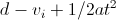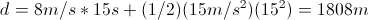### Example Question #8 : Motion In One Dimension

If a ball was thrown straight up at 10m/s and strikes the ground after two seconds, what maximum height did the ball reach?

15m

5m

7m

10m

2m

5m

Explanation:

This question can be solved using multiple strategies. One strategy uses the equation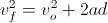.

vf = 0m/s

vo = 10m/s

a = -10m/s2

We use a negative acceleration because gravity is in the opposite direction of the movement of the ball. When we plug in all the values we find that d= 5m.

Another strategy uses the projectile time. In projectile motion we know that velocity is zero at the maximum height. Using only half of the projectile time, we can solve for the maximum height.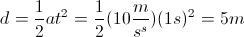### Example Question #9 : Motion In One Dimension

If a ball is thrown straight up into the air at a velocity of 20m/s and strikes the ground five seconds later, what is the velocity of the ball at its maximum height?

10m/s

7m/s

0m/s

5m/s

3m/s

0m/s

Explanation:

The velocity of the ball at the maximum height is equal to zero. Since the ball was thrown stright up, both the velocity in the x direction and the y direction are equal to zero at its peak. If the ball were thrown at an angle, only the velocity in the y direction would be equal to zero. This is a good point to keep in mind when doing kinematic problems. Note that there is still acceleration acting on the ball (gravity) of 10m/s2 which brings the ball back to the ground.

### Example Question #10 : Motion In One Dimension

If an object has an initial velocity of 3m/s and a constant acceleration of 2m/s2, how fast will the object be moving after five seconds?

15m/s

10m/s

7m/s

3m/s

13m/s

Using the equationwe can find the final velocity of the object after five seconds.